# Refractive properties of the α-BaGeO3 crystal and their origins: a density functional theory study†

Songming Wan*ab, Shengjie Jiang ac, Yu Zeng ac and Wen Luo a
aAnhui Provincial Key Laboratory of Photonics Devices and Materials, Anhui Institute of Optics and Fine Mechanics, Chinese Academy of Sciences, Hefei 230031, China. E-mail: smwan@aiofm.ac.cn
bState Key Laboratory of Advanced Special Steel, Shanghai University, Shanghai 200444, China
cUniversity of Science and Technology of China, Hefei 230026, China

Received 28th August 2020 , Accepted 22nd September 2020

First published on 22nd September 2020

Birefringent crystals are often applied to manipulate polarized states of light, yet the birefringent crystals used in the important mid-IR region are scarce. α-BaGeO3 is a potential mid-IR birefringent crystal due to its high mid-IR transmittance and strong structural anisotropy. Here, on the basis of density functional theory (DFT) calculations, we report the refractive characteristics of α-BaGeO3. The crystal is a positive biaxial crystal with the optical principal axes x, y and z parallel to the crystallographic b axis, 2.5° off the a axis, and 19.3° off the c axis, respectively. In the mid-IR region, the principle refractive indices nx, ny and nz are about 1.6297, 1.6308, and 1.6848, respectively. The maximum birefringence is about 0.055 which emerges when a light beam propagates along the x axis. These results indicate that the α-BaGeO3 crystal is a promising birefringent crystal used in the mid-IR region. Interestingly, α-BaGeO3 is quite similar to a uniaxial crystal in refractive characteristics, which is due to its unique layer structure. The refractive characteristics of α-BaGeO3 are associated with the Ba–O bonds; their spatial orientations determine the optical anisotropy of the crystal.

## 1 Introduction

Mid-IR polarized lasers have important applications in many fields, such as optics, analytical chemistry, molecular biology, biomedical diagnosis, and remote sensing.1–5 The birefringence observed in anisotropic crystals is often employed to create polarized lasers and control their polarized states.6 Most commercially available birefringent crystals, including CaCO3,7 α-BaB2O4,8 and YVO4,9 can only be used in the ultraviolet, visible, or near-infrared spectral region due to their poor transparency in the mid-IR region. On the other hand, many birefringent crystals transparent in the mid-IR region suffer from relatively small birefringences (e.g., MgF2 (ref. 10) and α-SiO2 (ref. 11)) or difficulty to obtain high-quality single crystals (e.g., TiO2).12 Thus, searching for new mid-IR birefringent crystals with better comprehensive performances is of great importance.

Our recent research revealed that low-temperature phase BaGeO3 (α-BaGeO3) is a mid-IR transparent crystal with an IR absorption edge extending to 5.9 μm. The crystal structure is alternately stacked by the layer of Ba2+ cations and the layer of [Ge3O9]6− six-membered rings along the crystallographic c axis.13 The layer structure is beneficial to generating strong optical anisotropy, and thus α-BaGeO3 is a potential mid-IR birefringent crystal. However, to the best of our knowledge, no investigation of its refractive and birefringent characteristics has been reported.

Traditionally, the refractive index and birefringence (the refractive index difference between two orthogonal polarized states) of a crystal are experimentally measured by using the minimum deviation method or the prism method.14,15 In parallel to the experimental method, density functional theory (DFT) calculation has recently emerged as a reliable method to predict the refractive index of crystal, in particular of a new crystal or the crystal temporarily difficult to be obtained.16,17 Compared with the experimental methods, the DFT method provides an indispensable approach to understand the origin of the refractive properties that is essential to rationally designing excellent birefringent crystals.14–18 In this paper, the DFT method was employed to investigate the refractive characteristics of the α-BaGeO3 crystal and to discuss their origins.

## 2 Computational methods

All of the DFT calculations were performed using the Cambridge Sequential Total Energy Package (CASTEP) code.19 The refractive indices of the Ba2MgGe2O7 crystal, which have been determined by the prism method,20,21 were used as the benchmark to test the performance of calculations at different theoretical levels. On the base of the test results, suitable calculation parameters were selected.

The reported Ba2MgGe2O7 and α-BaGeO3 crystal structures served as the initial structural model.13,20,21 The exchange–correlation functional was the revised Perdew–Burke–Ernzerhof (RPBE) formalism based on the generalized gradient approximation (GGA).22,23 Core–valence electron interactions were described using norm-conserving pseudopotentials.24 The valence electron configurations of composed atoms were 5s25p66s2 for barium, 2s22p63s2 for magnesium, 4s24p2 for germanium, and 2s22p4 for oxygen. The convergence criteria for the total energy change, maximum force, maximum stress, and maximum displacement were 10−5 eV per atom, 0.03 eV Å−1, 0.05 GPa, and 0.001 Å, respectively. After convergence tests, a k-point grid of 2 × 2 × 3 and an energy cutoff of 900 eV were selected for the Ba2MgGe2O7 crystal; a k-point grid of 2 × 2 × 2 and an energy cutoff of 800 eV for the α-BaGeO3 crystal (see the ESI, Table S1, Fig. S1, Table S2, and Fig. S2 for more details). The number of empty bands set for Ba2MgGe2O7/α-BaGeO3 was 1.5 times of that of valence bands to guarantee the calculation accuracy.15

The refractive index of Ba2MgGe2O7/α-BaGeO3 is obtained from its complex dielectric function:25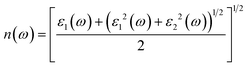(1)
where ε1(ω) and ε2(ω) are the real and imaginary parts of the complex dielectric function (ε(ω) = ε1(ω) + 2(ω)); ω is the incident light frequency.

The linear response expression of ε2(ω) is given by:25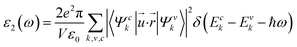(2)
where e is the electron charge; V is the unit cell volume; Ψck and Ψvk represent the wavefunctions of the conduction and valence bands at a given wavevector k, respectively; Eck and Evk are the energies of the conduction and valence bands, respectively;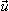is the vector defining the electric field of the incident light, and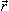is the position operator describing the electron transition.

ε 1(ω) can be derived from ε2(ω) using the Kramers–Kronig relation:25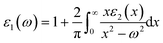(3)

Scissors operators were used in these calculations to compensate the underestimation of the GGA band gaps of Ba2MgGe2O7/α-BaGeO3.26 The GGA band gaps were given by the DFT method at the same theoretical level employed to investigate the refractive index. The experimental band gaps were obtained from the measured UV absorption edges. The absorption edge of Ba2MgGe2O7 reported by Becker et al. was adopted in this work.20 An α-BaGeO3 crystal grown from a high-temperature solution was cut and polished into a 4 mm thick transparent plate.13 The UV-vis transmittance spectrum of the α-BaGeO3 plate was recorded on a SolidSpec-3700 spectrophotometer in the wavelength range from 200 to 750 nm. From the spectrum, the UV absorption edge of α-BaGeO3 was obtained.

## 3 Results and discussion

Ba2MgGe2O7 is a positive uniaxial crystal. Its refractive indices have been experimentally determined in the wavelength range of 365–2325 nm by the prism method.20,21 In order to assess the reliability of the DFT method for calculating the refractive index of alkaline earth germanate crystals, we employed the DFT method to study the band structure and refractive dispersion curve of Ba2MgGe2O7. The calculated band gap is 3.678 eV (Fig. S3), smaller than the experimental value of 5.124 eV;21 thus a scissors operator of 1.446 eV was used to correct the calculated band gap. The calculated refractive dispersion curves of Ba2MgGe2O7 are shown in Fig. 1. The extraordinary ray has higher calculated refractive indices than the ordinary ray (ne > no), confirming the positive uniaxial nature of the crystal. Below 1.4 μm, the calculated refractive indices agree well with the experimental values. Above 1.4 μm, the calculated refractive indices are smaller than the experimental values because the phonon effect starts to manifest in the long-wave region.27 Nevertheless, the calculated birefringences (Δn = neno) are in accordance with the experimental results (with errors less than 5%). The above results demonstrate that the DFT method is reliable for predicting the refractive properties of alkaline earth germanate crystals, in particular their birefringent properties.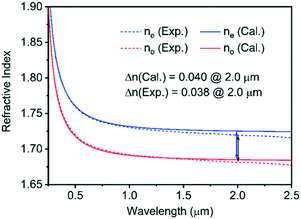Fig. 1 Experimental and calculated refractive dispersion curves of Ba2MgGe2O7.

The UV-vis transmittance spectrum of α-BaGeO3 is presented in Fig. 2. The UV absorption edge is located at 220 nm, corresponding to an experimental band gap of 5.636 eV. The calculated band structure indicates α-BaGeO3 is an indirect band material with a band gap of 3.622 eV (Fig. 2). Therefore, a scissors operator of 2.014 eV was applied to deal with the difference between the calculated and experimental band gaps. In addition, the refractive dispersion curve of α-BaGeO3 was studied with the incident light beam parallel to the crystallographic a axis. The results reveal the calculated refractive index almost invariable with the incident light wavelength in the mid-IR region (see Fig. S4 for more details); therefore, in the following sections, we only discuss the crystal refractive properties at 4 μm, a representative wavelength in the mid-IR region.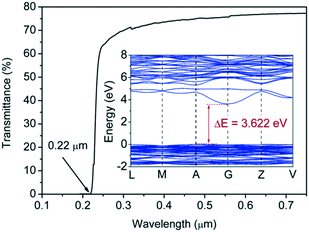Fig. 2 UV-vis transmittance spectrum and calculated band structure of α-BaGeO3.

The refractive index surface of an anisotropic crystal provides a straightforward way displaying the variation of refractive index with the propagation and polarized directions of an incident light beam.6 The refractive index surface has three mutually orthogonal principal axes (x, y, and z). For a monoclinic biaxial crystal, such as α-BaGeO3, one principal axis direction is along the crystallographic axis of twofold rotation symmetry, such as the b axis in the α-BaGeO3 unit cell.28 The other two principle axes are perpendicular to the twofold rotation axis, but not aligned with the other two crystallographic axes, such as the a and c axes in the α-BaGeO3 unit cell.

Since the ac plane of the α-BaGeO3 crystal is perpendicular to the b axis and thus a definite principle section, we first calculated no and ne in the ac plane by stepwise changing the incident light direction (10 degrees per step) and then calculated no and ne in the other two principle sections in a similar manner. The results are shown in Fig. 3. Under the convention of nx < ny < nz (nx = 1.6297, ny = 1.6308, and nz = 1.6848), we can conclude that the refractive index principal axes x, y and z are parallel to the crystallographic b axis, 2.5° off the a axis, and 19.3° off the c axis, respectively (Fig. 3d). The crystal is a positive biaxial crystal because nzny > nynx. The maximum birefringence is about 0.055 (Δn = nznx) which appears when the incident light propagates along the x axis. The moderate birefringence indicates that α-BaGeO3 is a promising mid-infrared birefringent crystal.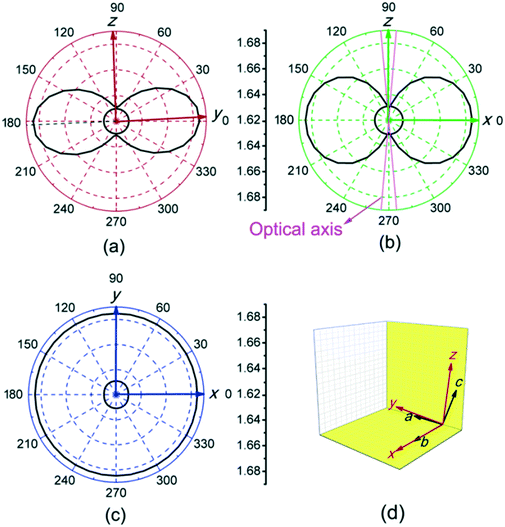Fig. 3 (a) yz, (b) xz, and (c) xy principle sections of the α-BaGeO3 refractive index surface; (d) relations between the crystallographic axes (a, b and c) and the refractive index principal axes (x, y and z).

It is interesting that, although α-BaGeO3 is a biaxial crystal, it is quite similar to a uniaxial crystal in refractive characteristics. For example, its principal refractive indices nx (1.6297@4 μm) and ny (1.6308@4 μm) are very close; its two optic axes almost coincide (both are about 5° off the z-axis, as shown in Fig. 3b). The unique refractive characteristics are favourable for manufacturing birefringent devices from α-BaGeO3 as compared with other biaxial crystals, such as BaTeMo2O9 (ref. 29) and BaTeW2O9.30 These characteristics arise from its special crystal structure. The α-BaGeO3 crystal is stacked by alternate [Ge3O9]6− and Ba2+ layers along the z axis.13 Ba2+ and [Ge3O9]6− are arranged in a near threefold rotation symmetry in their own planes (Fig. 4a and b); thus, like the cases of the crystal in the trigonal system, the properties of α-BaGeO3, including the refractive properties, are almost the same in all directions perpendicular to the z axis.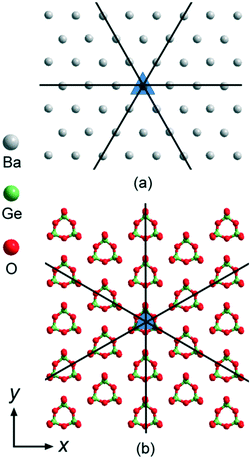Fig. 4 (a) Ba2+ and (b) [Ge3O6]6− layers of α-BaGeO3 viewed down the z axis.

The electronic states play a primary role in the analysis of the refractive properties of α-BaGeO3.31 The total and partial densities of states (TDOS and PDOS) of α-BaGeO3 are shown in Fig. 5. The valence bands lower than −9.0 eV mainly originates from Ba 5s, Ba 5p, and O 2s states mixing with minor Ge 4s and Ge 4p states. The bands between −9.0 eV and the Fermi level are composed of O 2p states with a small portion of Ge 4s and Ge 4p states. The bottom of the conduct band is dominated by Ba 5d states with partly contribution of Ba 6s, Ge 4s, Ge 4p and O 2p states.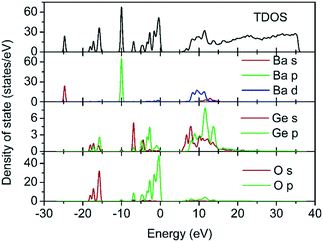Fig. 5 TDOS and PDOS of α-BaGeO3.

Since n(ω) is positively correlated to ε2(ω) in the crystal transparent region (eqn (1) and (3)), ε2(ω) is the essential factor in determining n(ω). Fig. 6 shows the imaginary parts of α-BaGeO3 (ε2(x), ε2(y) and ε2(z)) along the three refractive index principal axes (x, y and z) at the fixed incident light wavelength of 4 μm. When the light energy is less than the band gap of α-BaGeO3, the ε2 value is equal to zero. When the energy is larger than the band gap, at least eight peaks are present in the ε2 curves. In accordance with the above DOS analyses, peaks in region I (7.5–17.0 eV) correspond to the electron transitions from the O 2p to Ba 5d states; peaks in region II (17.0–22.5 eV) arise from the electron transitions between the Ba 5p and Ba 5d states. According to eqn (1) and (3), the contribution from the O-to-Ba transitions is more significant to ε2 and thus the refractive properties of α-BaGeO3 are primarily associated with the Ba–O bonds.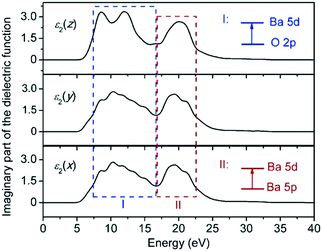Fig. 6 Imaginary parts of the dielectric functions of α-BaGeO3 along the three refractive index principal axes.

The imaginary part ε2(ω) of the dielectric function is positively correlated to the transition matrix element 〈Ψck|·|Ψvk〉 (eqn (2)). For a fixed couple of Ψck and Ψvk, the value of the transition matrix element is determined by·. According to the above analyses, the electron transition vectors () are along the directions of the Ba–O bonds, and thus the angles between the Ba–O bonds and the polarized direction of the incident light () determine the value of·. The α-BaGeO3 crystal contains two crystallographically distinct Ba2+ cations, both of which are surrounded by eight oxygen atoms. Six oxygen atoms (O1) among them are arranged in the symmetrical form of pseudo-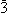(theaxis is parallel to the z axis), and all of the Ba–O1 bonds lean to the xy plane at a nearly identical angle (Fig. 7(a)). The other two oxygen atoms (O2) form two Ba–O2 bonds tilting around the z axis. The spatial orientations of these Ba–O bonds means that·, together with ε2(ω), ε1(ω) and n(ω), has an approximately equal value whenlies in the xy plane and a different value whenis perpendicular to the xy plane, which is consistent with the calculated results shown in Fig. 3. The slight difference in refractive index along the x- and y-axes is due to the slight difference in the tilt angles of the Ba–O2 bonds to the two axes (Fig. 7).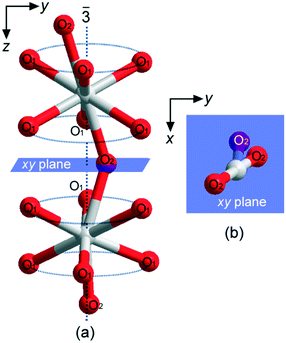Fig. 7 Ba–O bonds in the α-BaGeO3 crystal structure viewed along (a) the x-axis and (b) z-axis.

## Conclusions

The DFT method was used to investigate the refractive properties of the α-BaGeO3 crystal. Its refractive index principal axes x, y and z are parallel to the crystallographic b axis, 2.5° off the a axis, and 19.3° off the c axis, respectively. α-BaGeO3 is a positive biaxial crystal with nx = 1.6297@4 μm, ny = 1.6308@4 μm, and nz = 1.6848@4 μm. The maximum birefringence is about 0.055 which appears when the incident light beam propagates along the x axis (the crystallographic b axis). The results indicate that α-BaGeO3 is a promising birefringent crystal used in the mid-IR region. α-BaGeO3 is quite similar to a uniaxial crystal in refractive properties because [Ge3O9]6− and Ba2+ are arranged in a near threefold rotation symmetry in their own planes. The refractive properties of α-BaGeO3 are primarily associated with the electron transitions between the O 2p and Ba 5d states and the spatial orientations of the Ba–O bonds. We believe these theoretical insights to be helpful for exploring new birefringent crystals.

## Conflicts of interest

There are no conflicts to declare.

## Acknowledgements

The work is financially supported by The National Natural Science Foundation of China (Grant No. 51372246), Open Project of State Key Laboratory of Advanced Special Steel, Shanghai University, and Open Project of Key Laboratory of Functional Crystals and Laser Technology, TIPC, CAS. The calculations were partially performed at the Center for Computational Science, CASHIPS.

## Notes and references

1. A. Lakhtakia, Selected Papers on Natural Optical Activity, SPIE Press, Bellingham, WA, USA, 1990 Search PubMed.
2. J. S. Tyo, D. L. Goldstein, D. B. Chenault and J. A. Shaw, Appl. Opt., 2006, 45, 5453–5469 Search PubMed.
3. F. Cremer, W. D. Jong and K. Schutte, Opt. Eng., 2002, 41, 1021–1032 Search PubMed.
4. I. Yamada, N. Yamashita, K. Tani, T. Einishi, M. Saito, K. Fukumi and J. Nishii, Opt. Lett., 2011, 36, 3882–3884 Search PubMed.
5. H. Cheng, S. Q. Chen, P. Yu, J. X. Li, B. Y. Xie, Z. C. Li and J. G. Tian, Appl. Phys. Lett., 2013, 103, 223102 Search PubMed.
6. G. Chartier, Introduction to Optics, Springer, New York, NY, USA, 2005 Search PubMed.
7. R. E. Nyswander, Phys. Rev., 1909, 28, 291–308 Search PubMed.
8. R. Appel, C. D. Dyer and J. N. Lockwood, Appl. Opt., 2002, 41, 2470–2480 Search PubMed.
9. H. T. Luo, T. Tkaczyk, E. L. Dereniak, K. Oka and R. Sampson, Opt. Lett., 2006, 31, 616–618 Search PubMed.
10. M. J. Dodge, Appl. Opt., 1984, 23, 1980–1985 Search PubMed.
11. G. Ghosh, Opt. Commun., 1999, 163, 95–102 Search PubMed.
12. J. R. Devore, J. Opt. Soc. Am., 1951, 41, 416–419 Search PubMed.
13. S. M. Wan, Y. Zeng, Y. N. Yao, M. R. D. Mutailipu, J. Han, S. J. Jiang, S. J. Zhang and S. L. Pan, Inorg. Chem., 2020, 59, 3542–3545 Search PubMed.
14. H. P. Wu, S. L. Pan, H. W. Yu, D. Z. Jia, A. M. Chang, H. Y. Li, F. F. Zhang and X. Huang, CrystEngComm, 2011, 14, 799–803 Search PubMed.
15. X. L. Chen, B. B. Zhang, F. F. Zhang, Y. Wang, M. Zhang, Z. H. Yang, K. R. Poeppelmeier and S. L. Pan, J. Am. Chem. Soc., 2018, 140, 16311–16319 Search PubMed.
16. Y. Zhong, P. Shan, T. Q. Sun, Z. P. Hu, H. D. Liu, S. G. Liu, Y. F. Kong and J. J. Xu, CrystEngComm, 2019, 21, 4690–4695 Search PubMed.
17. M. A. F. Afzal and J. Hachmann, Phys. Chem. Chem. Phys., 2019, 21, 4452–4460 Search PubMed.
18. C. S. Lin, A. Y. Zhou, W. D. Cheng, N. Ye and G. L. Chai, J. Phys. Chem. C, 2019, 123, 31183–31189 Search PubMed.
19. V. Milman, K. Refson, S. J. Clark, C. J. Pickard, J. R. Yates, S. P. Gao, P. J. Hasnip, M. I. J. Probert, A. Perlov and M. D. Segall, J. Mol. Struct., 2010, 954, 22–35 Search PubMed.
20. P. Becker, P. Held, J. Liebertz and L. Bohaty, Cryst. Res. Technol., 2010, 44, 603–612 Search PubMed.
21. A. A. Kaminskii, L. Bohaty, P. Becker, J. Liebertz, P. Held, H. J. Eichler, H. Rhee and J. Hanuza, Laser Phys. Lett., 2010, 5, 845–868 Search PubMed.
22. B. Hammer, L. B. Hansen and J. K. Norskov, Phys. Rev. B: Condens. Matter Mater. Phys., 1999, 59, 7413–7421 Search PubMed.
23. J. P. Perdew, K. Burke and M. Ernzerhof, Phys. Rev. Lett., 1996, 77, 3865–3868 Search PubMed.
24. D. R. Hamann, M. Schlüter and C. Chiang, Phys. Rev. Lett., 1979, 43, 1494–1497 Search PubMed.
25. D. W. Lynch, in Handbook of Optical Constants of Solid, ed. E. D. Palik, Academic Press, San Diego, CA, USA, 1997 Search PubMed.
26. S. Baroni, S. Gironcoli, A. D. Corso and P. Giannozzi, Rev. Mod. Phys., 2001, 73, 515–562 Search PubMed.
27. R. C. Xiao, H. X. Wu, Y. B. Ni, Z. Y. Wang, C. B. Huang, M. Qi and C. W. Ge, J. Appl. Phys., 2015, 117, 135702 Search PubMed.
28. S. Alexander, P. Yurii and K. Bart, Optically Anomalous Crystals, Springer, Berlin, Germany, 2007 Search PubMed.
29. Q. X. Yu, Z. L. Gao, S. J. Zhang, W. G. Zhang, S. P. Wang and X. T. Tao, J. Appl. Phys., 2012, 111, 013506 Search PubMed.
30. Z. H. Zhang, X. T. Tao, J. J. Zhang, Y. X. Sun, C. Q. Zhang and B. Li, CrystEngComm, 2013, 15, 10197–10204 Search PubMed.
31. M. R. D. Mutailipu, M. Zhang, B. B. Zhang, Z. H. Yang and S. L. Pan, Chem. Commun., 2018, 54, 6308–6311 Search PubMed.

### Footnote

Electronic supplementary information (ESI) available: Convergence tests for the total energies of the Ba2MgGe2O7 and α-BaGeO3 crystals with respect to their Γ-centred k-point grids and kinetic energy cutoffs; calculated band structure of Ba2MgGe2O7; calculated refractive dispersion curves of α-BaGeO3 (PDF). See DOI: 10.1039/d0ce01265c
 This journal is © The Royal Society of Chemistry 2020